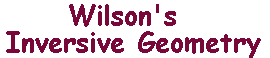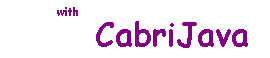Stereographic Projection - an alternative approach to the extended plane and i-lines

 The material on these pages gives an alternative approach to the introduction of the point at infinity and the concepts of extended lines and i-lines. It is not used to prove any results not proved elsewhere. Since the World is approximately spherical, geographers have considered the problem of representing the surface on a plane. The easiest solution is to use a projection map. There are many ways to do this. We shall look at stereographic projection. Definition Let S be a sphere with centre O, and let N be a point on S. let P be a plane normal to NO, not through N. For a point P on S other than N, the stereographic projection of P with vertex N onto P is the point Q where NP meets P. We denote it by p(P). In the sketch, we have taken N as the North Pole of the sphere, so that P is parallel to the Equator. Note that the changing P merely changes the scale of the resulting picture since the image Q always lies on NP. If we choose Q on P, then the line NQ cuts S-{N} exactly once. Thus p is one-one and maps S-{N} onto P.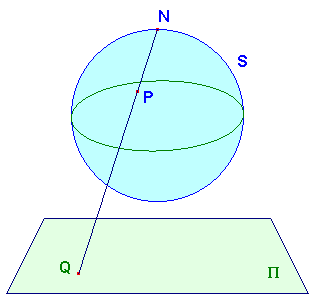The map p is so far defined only on S -{N}. To extend it to all of S, we introduce the extended plane P+=P+{Ñ}, where Ñ is called the point at infinity. We say that p(N) = Ñ. Now p gives a bijection from S to P+{Ñ}. The inverse p -1 maps P to S-{N}, and Ñ to N. See The Extended Plane for an alternative approach Circles on S Let F be a plane which cuts S non-trivially. Then F meets S in a circle C. You will find a proof here. Since a circle is a plane figure, every circle on S arises in this way. Although we shall not pursue it far, there is a geometry on S which has as its lines the circles on S. We shall, however, show that angles can be defined in this geometry. If P is a point on C, then there is a tangent to C at P. This lies in F.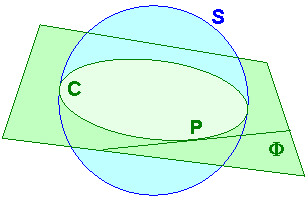Definition If C and D are circles on S meeting in P, then the angle between C and D at P is the angle between the tangents at P. Of course, the circles will in general meet again, at Q say. Think of reflecting in the plane bisecting PQ and normal to PQ. This interchanges P and Q and the tangent pairs, so the angles at P and Q are of equal magnitude. If we view the picture looking in towards the centre of the sphere, we may attach a sense to angles. Since the above proof used areflection, we see that the angles at P and Q have opposite sense. We now prove two theorems which together show that this geometry on S is actually a model of inversive geometry!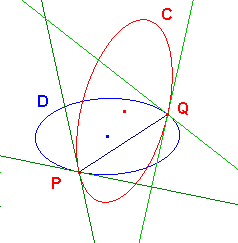Theorem 1 Let p denote stereographic projection with vertex N from the sphere S to the plane P. Then, for any circle C on S, p(C) is a line if N lies on C, and a circle otherwise. Theorem 2 Stereographic projection preserves angles. Proof of Theorem 2